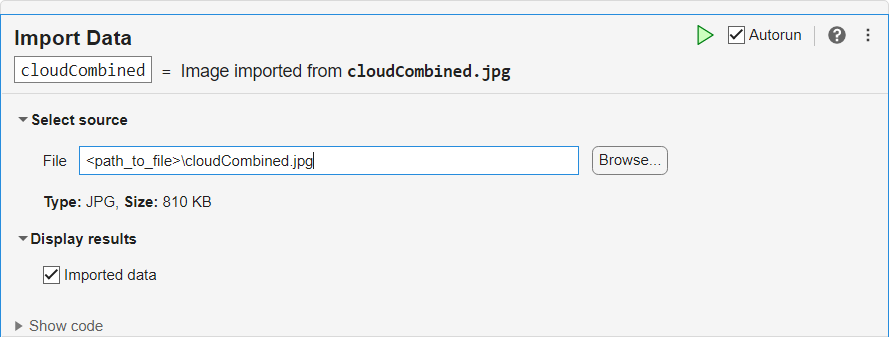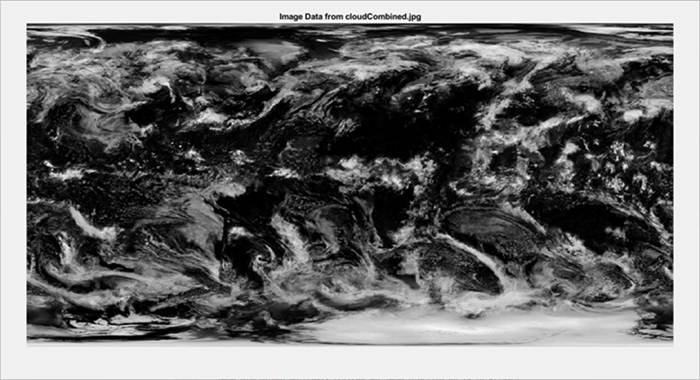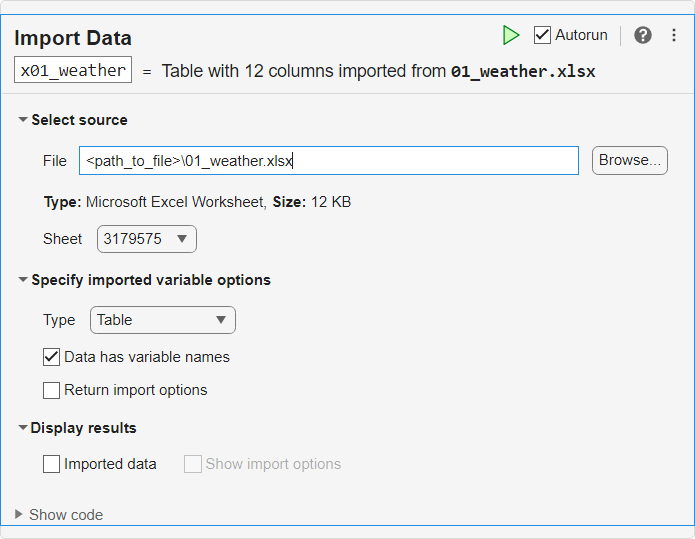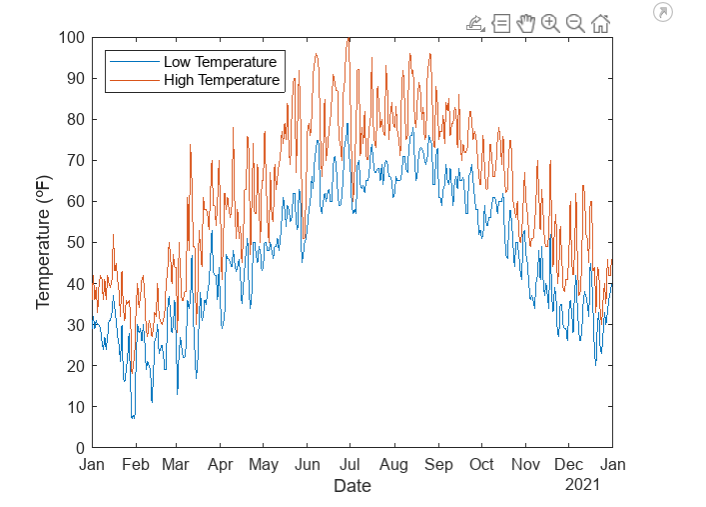# Import Data

Import data from a file in the Live Editor

Since R2023a

## Description

The Import Data task lets you interactively import MAT, text, spreadsheet, media (image, audio, and video), and scientific data (netCDF) files. The task automatically generates MATLAB® code for your live script.

To add the Import Data task to a live script in the MATLAB Editor:

• On the tab, select .

• In a code block in the script, type a relevant keyword, such as `import`, `read`, or `load`. Select `Import Data` from the suggested command completions.

## Examples

expand all

Interactively import data from an image file.

Open the Import Data task in the Live Editor. Select the source file and then select the Imported data option in the Display results section to show the image. The task saves the image data in a variable in the workspace. The variable name is based on the name of the source file.To see the code that this task generates, expand the task display by clicking at the bottom of the task parameter area.

```% Import image cloudCombined = imread("<path_to_file>\cloudCombined.jpg"); % Display results imshow(cloudCombined); title("Image Data From cloudCombined.jpg");```Interactively generate reusable MATLAB code to import a Microsoft® Excel® spreadsheet into your workspace. The spreadsheet contains weather data for Boston, Massachusetts, for the month of January 2021. Use the generated code as a template to programmatically import spreadsheets for all twelve months of 2021.

Open the Import Data task in the Live Editor. Enter the filename of the first spreadsheet, `01_weather.xlsx`, in the File field.To see the code that this task generates, expand the task display by clicking at the bottom of the task parameter area.

```% Set up the Import Options and import the data opts = spreadsheetImportOptions("NumVariables", 12); % Specify sheet and range opts.Sheet = "3179575"; opts.DataRange = "A2:L32"; % Specify column names and types opts.VariableNames = ["STATION", "NAME", "LATITUDE", "LONGITUDE", "ELEVATION", "DATE", "AWND", "PRCP", "SNOW", "TAVG", "TMAX", "TMIN"]; opts.VariableTypes = ["categorical", "categorical", "double", "double", "double", "datetime", "double", "double", "double", "double", "double", "double"]; % Specify variable properties opts = setvaropts(opts, ["STATION", "NAME"], "EmptyFieldRule", "auto"); % Import the data x01_weather = readtable("<path_to_file>\01_weather.xlsx", opts, "UseExcel", false); % Clear temporary variables clear opts```

The generated code is separated into steps and documented with comments. Make these changes to automate the process of importing all twelve monthly spreadsheets:

1. Create a `yearWeather` table, to which you can append the data from each of the monthly spreadsheets.

2. Create a `namePrefixes` vector that contains strings representing each month.

3. Create a vector of filenames using `namePrefixes`. Use the regular pattern of the twelve filenames.

4. Create a vector of data ranges based on the number of days in each month. In the generated code, the range spans columns A to L and rows 2 to 32 (one more than the number of days in January).

5. Use a `for`-loop and the variables you created to import all files and append them in `yearWeather`.

```% Changes #1 and #2: Create variables yearWeather = table(); namePrefixes = ["01" "02" "03" "04" "05" "06" "07" "08" "09" ... "10" "11" "12"]; % Set up the Import Options and sheet opts = spreadsheetImportOptions("NumVariables", 12); opts.Sheet = "3179575"; % Specify column names and types opts.VariableNames = ["STATION", "NAME", "LATITUDE", "LONGITUDE", ... "ELEVATION", "DATE", "AWND", "PRCP", "SNOW", ... "TAVG", "TMAX", "TMIN"]; opts.VariableTypes = ["categorical", "categorical", "double", ... "double", "double", "datetime", "double", ... "double", "double", "double", "double", "double"]; % Specify variable properties opts = setvaropts(opts, ["STATION", "NAME"], "EmptyFieldRule", "auto"); % Changes #3 and #4: Create vectors of filenames and data ranges fileNames = namePrefixes + "_weather.xlsx"; dataRanges = "A2:L"+string(day(datetime(2021,1,31)+calmonths(0:11))+1); % Change #5: Import all files and append them in yearWeather for i = 1:length(namePrefixes) fileName = fileNames(i); opts.DataRange = dataRanges(i); monthWeather = readtable(fileName, opts, "UseExcel", false); yearWeather = [yearWeather; monthWeather]; end % Clear temporary variables clear opts```

Use the imported data to plot the daily low and high temperatures in Boston for each day of 2021.

```plot(yearWeather.DATE,[yearWeather.TMIN yearWeather.TMAX]) hold on xlabel("Date") ylabel("Temperature (℉)") legend("Low Temperature","High Temperature",location="Northwest")```## Parameters

expand all

Select source

You can enter the path or use the button. The type of the file that you choose to import determines the other parameters in that task that you can select.

When importing a spreadsheet file, select the sheet to import.

Specify imported variable options

When importing a text file or spreadsheet, you can choose the MATLAB data type of the imported data:

• `Table` — Data is imported as a MATLAB table. Each column of the data is a variable of the table, and each row of the data is a row of the table. Variable names of the table are derived from column headers of the data.

• `Timetable` — Data is imported as a MATLAB timetable. If the first column of the data is not of type `datetime` or `duration`, then a new first column of `duration` values is prepended to the table that starts at 0 s and increments by 1 s for each row of the data.

• `Column vectors` — Data is imported as MATLAB column vectors. Each column of the data becomes a column vector, and the name of each column vector variable is derived from the header of the corresponding column of the data.

• `Numeric array` — Data is imported as a numeric matrix.

• `String array` — Data is imported as a string matrix.

• `Cell array` — Data is imported as a cell matrix.

If you select this option, then the task preserves the import options in a variable named `opts` by default. If you do not select this option, then the `opts` variable is cleared after the import is complete. This parameter is available when importing a text file or spreadsheet.

Display results

Select this option to show the imported data as output of your Live Editor task.

If you select this option, then your import options appear as output of your Live Editor task. If you do not select this option, then the options do not appear. This parameter is available when importing a text file or spreadsheet.

## Version History

Introduced in R2023a

expand all